International
Tables for
Crystallography
Volume C
Mathematical, physical and chemical tables
Edited by E. Prince

International Tables for Crystallography (2006). Vol. C, ch. 8.2, p. 689

## Section 8.2.1. Maximum-likelihood methods

E. Princea and D. M. Collinsb

aNIST Center for Neutron Research, National Institute of Standards and Technology, Gaithersburg, MD 20899, USA, and bLaboratory for the Structure of Matter, Code 6030, Naval Research Laboratory, Washington, DC 20375-5341, USA

### 8.2.1. Maximum-likelihood methods

| top | pdf |

In Chapter 8.1, structure refinement is presented as finding the answer to the question, given a set of observations drawn randomly from populations whose means are given by a model, M(x), for some set of unknown parameters, x, how can we best determine the means, variances and covariances of a joint probability density function that describes the probabilities that the true values of the elements of x lie in certain ranges?'. For a broad class of density functions for the observations, the linear estimate that is unbiased and has minimum variances for all parameters is given by the properly weighted method of least squares. The problem can also be stated in the slightly different manner, given a model and a set of observations, what is the likelihood of observing those particular values, and for what values of the parameters of the model is that likelihood a maximum?'. This set of parameters is the maximum-likelihood estimate.

Suppose the ith observation is drawn from a population whose p.d.f. is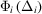, where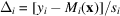, x is the set of `true' values of the parameters, and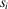is a measure of scale appropriate to that observation. If the observations are independent, their joint p.d.f. is the product of the individual, marginal p.d.f.s: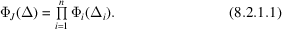The functioncan also be viewed as a conditional p.d.f. forgiven, or, equivalently, as a likelihood function for x given an observed value of, in which case it is written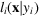. Because a value actually observed logically must have a finite, positive likelihood, the density function in (8.2.1.1)and its logarithm will be maximum for the same values of x: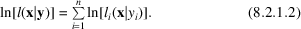In the particular case where the error distribution is normal, and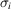, the standard uncertainty of the ith observation, is known, then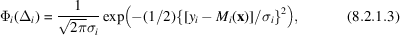and the logarithm of the likelihood function is maximum when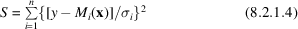is minimum, and the maximum-likelihood estimate and the least-squares estimate are identical.

For an error distribution that is not normal, the maximum-likelihood estimate will be different from the least-squares estimate, but it will, in general, involve finding a set of parameters for which a sum of terms like those in (8.2.1.2)is a maximum (or the sum of the negatives of such terms is a minimum). It can thus be expressed in the general form: find the minimum of the sum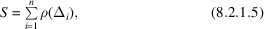where ρ is defined by ρ(x) = −ln [Φ(x)], and Φ(x) is the p.d.f. of the error distribution appropriate to the observations. If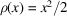, the method is least squares. If the error distri­bution is the Cauchy distribution,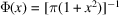,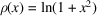, which increases much more slowly than x2 as |x| increases, causing large deviations to have much less influence than they do in least squares.

Although there is no need for ρ(x) to be a symmetric function of x (the error distribution can be skewed), it may be assumed to have a minimum at x = 0, so that dρ(x)/dx = 0. A series expansion about the origin therefore begins with the quadratic term, and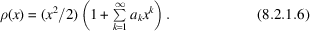This procedure is thus equivalent to a variant of least squares in which the weights are functions of the deviation.